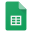# IMPORTRANGE with QUERY

### In this example we show how to use IMPORTRANGE within a QUERY to match values.

This is the main inventory sheet that can be referenced with importrange to match data here with data collected from a form. Both these sheets are shared so anyone can edit. Pop open the sheets and see how it works.InventoryCell A1 has a list of all values in Column H (SORT(UNIQUE(H2:H)) which is then used as a data validation drop down list. when you choose a value, QUERY(IMPORTRANGE()) pulls the values from the main inventory sheet.

In this sheet we use this formula:

• `=QUERY(IMPORTRANGE("https://docs.google.com/spreadsheets/d/1mYZ1DjmJMJkvFM50e_s2LCeDjk5rpIQpW1gG61cB2ps","All!A4:H"), "select * where Col8 ='"&A1&"'")`
• This formula queries via importrange and returns the rows of data where the data validation value in cell A1 match the Column H "Col8".
• This can be used to match information submitted on a form with other data that one has on a different sheet.
• Modify the formula to query a different row for a different set of values.Katie example